# Application of Least Squares Meshless Method in Multi-component High-Speed Non-equilibrium Reaction Jet

Application of Least Squares Meshless Method in Multi-component High-Speed Non-equilibrium Reaction Jet

Liang WangRui Xue Ning Cai Wei Wu Miaomiao Niu Dongliang Zhang

School of Energy and Power Engineering, Nanjing Institute of Technology, Nanjing 211167, China

Beijing Aerospace Technology Research Institute, Beijing 100074, China

Corresponding Author Email:
wl_njit@njit.edu.com
Page:
507-514
|
DOI:
https://doi.org/10.18280/ijdne.150407
7 March 2020
|
Accepted:
13 June 2020
|
Published:
31 August 2020
| Citation

OPEN ACCESS

Abstract:

This paper designs a novel least squares (LS) meshless method for the three-dimensional (3D) problem of multi-component high-speed non-equilibrium reaction jet. Specifically, the space derivative was solved by the LS method, and the artificially upstream flux vector splitting (AUFS) scheme was introduced to compute the convection term in the control equation. Considering the possibility of chemical reactions, the flow field parameters were updated by the finite-rate reaction model; the rigidity of chemical reactions was solved by the time splitting method. In addition, the 3D computational domain was discretized into simple weighted points with clear physical meanings. On this basis, the complex problems of the steady flow around the high-speed flying projectile with the angle of attack, and the 3D muzzle flow field with brake were calculated. The results prove that the proposed LS meshless method is a feasible way to capture the shock structure in complex flow fields. The proposed method provides a new solution to multi-component high-speed non-equilibrium reaction jets.

Keywords:

least squares (LS), meshless method, multi-component chemical reaction, artificially upstream flux vector splitting (AUFS) scheme

1. Introduction

Multi-component high-speed non-equilibrium reaction jets are widely used in aviation, aerospace, weapons, and other fields. The non-equilibrium flow formed by the external high temperature environment of hypersonic aircraft, the supersonic combustion flow in the propulsion system of aircraft, the re-entry of aircraft into the atmosphere, and gunpowder gas flow of gun muzzle, etc., have always been the focus and difficulty in computational fluid dynamics. The difficult points in the multi-component high-speed non-equilibrium reaction jet mainly include the complex physical process, the rigidity of chemical reaction [1-3] and the huge amount of calculation.

The rigidity problem of chemical reaction calculation is the most difficult to solve. The chemical reaction rate is extremely sensitive to the thermodynamic state function, resulting in a large difference in the characteristic time scale between different reactions; the reaction characteristic time scale is also several orders of magnitude smaller than the flow characteristic time scale. Splitting and non-splitting are currently the two mainstream methods to solve the decoupling of flow and chemical reaction. Non-splitting method can be divided into point implicit algorithm and fully implicit algorithm. Fedkiw et al.  used the second-order Strange splitting method to solve the N-S equation with reaction source terms, and Newton-Raphson iteration to update the temperature. da Silva et al.  adopted the time splitting method to simulate the oblique detonation wave stationary on the wedge. Liu et al.  proposed a new time splitting method to solve the non-equilibrium chemical reaction flow, and used a gradient formula to solve the chemical reaction source term, with a high calculation efficiency. Saghafian et al.  calculated the multi-component high-speed non-equilibrium reaction jet using a model based on a variable flame surface; the chemical model used three scalar equations, performing well in large eddy simulation calculations. Mei et al.  studied the chemical reaction of the reentry flow field of a supersonic aircraft, established a gas phase chemical reaction model and a wall reaction model, and achieved the calculation results of different combinations. Santillana et al.  conducted research on atmospheric chemistry models, verifying the influence of the order of operator splitting on the accuracy of decoupling methods. Bussing and Murmanl  first applied the point implicit algorithm to unify the time steps of chemical reactions and convection terms to pseudo time steps for solving the rigidity problem of the equation. Lee et al.  simulated the non-equilibrium supersonic flow based on a Cartesian grid in the same method. Sussman  introduced a scale factor to modify the chemical reaction source term, and adopted a point-hidden algorithm to simulate the shock-induced oscillating combustion flow field. Shuen and Yoon  put forward the LU-SSOR format, and conducted implicit computation of convection terms and reaction source terms, while explicitly processing the viscosity terms to reduce the amount of calculation. Sun et al.  combined the dynamic multi-time scale of Gou et al.  with the dynamic adaptive chemical process to form the HMTS/CDAC method, thereby improving the computational efficiency significantly.

In traditional computational fluid dynamics (CFD), grid-based finite difference method (FDM), finite element method (FEM), and finite volume method (FVM) are more widely used, but the existence of grids leads to certain limitations in the use of certain flow fields [16-18]. To solve this, Perrone and Kao ; Liszka and Orkisz  established the prototype of a meshless algorithm in the 1970s. The meshless algorithm [21-23] breaks through the limitations of grid division, and adopts the discrete nodes distributed in the boundary or within the computational domain, showing a huge advantage in analyzing the extremely large deformations and serious deformities of the multi-media, fluid-solid coupling, etc. Löhner et al.  and Ortega et al. , based on the finite point method, used Taylor–Galerkin format to successfully solve 2D and 3D compressible flows. Erhart et al.  developed a localized and virtual radial basis function, and then proposed a local meshless method based on the Cartesian grid, which successfully solved the problem of supersonic flow around flat plat. Munikrishna and Balakrishnan  applied a hybrid meshless-Cartesian grid method to calculate turbulent flow using the RANS turbulence model coupled with the zero equation of the meshless solver LSFD-U. Gargari et al. [28, 29] proposed a mixed discrete least squares meshless (MDLSM) method for the incompressible Navier-Stokes equation and ALE equation, enjoying higher accuracy than the DLSM method.

This paper attempts to present a LS meshless method for numerical simulation of 3D multi-component high-speed non-equilibrium reactive jets. First, the 3D multi-component control equations and numerical methods used were briefly introduced, and then the flow field was discretized using the weighted points. Finally, numerical simulation was conducted on the steady flow around the high-speed flying projectile with the angle of attack, and the 3D muzzle flow field with brake. The results are in good agreement with the experiment and two-dimensional calculation results, proving the effectiveness of the algorithm.

2. Control Equations and Numerical Methods

In the 3D non-equilibrium chemical reaction flow, the flow field can be described by the 3D Euler equation with the reaction source term. It’s expressed in the rectangular coordinate system as:

$U_{t}+F_{1, x}+F_{2, y}+F_{3, z}=S$       (1)

where, U is a conserved variable, F1, F2, and F3 are convective fluxes, and S is a chemical reaction source term. Specifically, they’re expressed as:

$U=\left(\begin{array}{llllllll}\rho_{1} & \rho_{2} & \cdots & \rho_{N_{R}} & \rho u & \rho v & \rho_{w} & \rho E\end{array}\right)^{T}$

$F_{1}=\left(\begin{array}{llllllll}\rho_{1} u & \rho_{2} u & \cdots & \rho_{N_{k}} u & \rho u u+p & \rho v u & \rho w u & \rho E u+u p\end{array}\right)^{T}$

$F_{2}=\left(\begin{array}{lllllllllllll}\rho_{1} v & \rho_{2} v & \cdots & \rho_{N_{R}}v & \rho u v+p & \rho v v & \rho w v & \rho E v+v p\end{array}\right)^{T}$   (2)

$F_{3}=\left(\begin{array}{lllllllll}\rho_{1} w & \rho_{2} w & \cdots & \rho_{N_{R}} w & \rho u w+p & \rho v w & \rho w w & \rho E w+w p\end{array}\right)^{T}$

$S=\left(\begin{array}{llllllll}\omega_{1} & \omega_{2} & \cdots & \omega_{N_{R}} & 0 & 0 & 0 & 0\end{array}\right)^{T}$

where, ρi is the mass density of component i, NR is the total number of components, ρ is the mass density of the mixed gas, u, v, and w are the velocity components in the x, y, and z directions of the mixed gas, p is the pressure of the mixed gas, ωi is the mass production rate of component i caused by chemical reaction, and ρE is the total energy per unit volume.

$\omega_{i}=\sum_{t=1}^{R_{N}}\left(\nu_{i t}^{R}-v_{i t}^{L}\right)\left[K_{f t}\prod_{k=1}^{N_{R}}\left(\frac{\rho_{k}}{M_{k}}\right)_{k}^{v_{k t}^{L}}-K_{b t}\prod_{k=1}^{N_{R}}\left(\frac{\rho_{k}}{M_{k}}\right)^{v_{k t}^{R}}\right] \cdot M_{i}$      (3)

In the formula (3), $v_{i t}^{L}, v_{i t}^{R}$ are the equivalent coefficients of the chemical reaction on the left and right side of the component i under the reaction t; Mi is the molar mass of the component t; RN is the total number of chemical reactions in the model; Kft is the forward and reverse reaction rate constant, calculated by the Arrhenius formula:

$K_{f t}=A_{t} T^{b_{t}} \exp \left(-\frac{E_{t}}{R_{u} T}\right)$       (4)

Among them, At is thepre-exponential factor, bt is the temperature factor, Et is the activation energy, Ru is the general gas constant, and T is the mixed gas temperature. The reverse reaction rate constant can be calculated from the reaction equilibrium constant, and the chemical reaction source term is processed by the finite rate reaction model .

2.1 Space derivative

In the 3D case, it’s assumed that the flow variable satisfies the following linear function relationship:

$f=a_{1} x+a_{2} y+a_{3} z+a_{0}=\frac{\partial f}{\partial x} x+\frac{\partial f}{\partial y} y+\frac{\partial f}{\partial z} z+a_{0}$      (5)

In the point-cloud structure of any node i, the flow variables of the center point i and its satellite points j(j=1,2,…,N) all satisfy the equation above, and then the following matrix can be given as:

$\left(\begin{array}{cccc}\frac{x_{1}+x_{i}}{2} & \frac{y_{1}+y_{i}}{2} & \frac{z_{1}+z_{i}}{2} & 1 \\ \vdots & \vdots & \vdots & \vdots \\ \frac{x_{N}+x_{i}}{2} & \frac{y_{N}+y_{i}}{2} & \frac{z_{N}+z_{i}}{2} & 1\end{array}\right) \cdot\left(\begin{array}{l}a_{1} \\ a_{2} \\ a_{3} \\ a_{0}\end{array}\right)=\left(\begin{array}{c}\frac{f_{1}+f_{i}}{2} \\ \vdots \\ \frac{f_{N}+f_{i}}{2}\end{array}\right)$       (6)

Given that the coefficient matrix of the above formula is A, generally, the number of satellite points of a node is over 4, so the above is a contradiction equation. The space derivative at any node i is obtained by the LS method:

$\left.\frac{\partial f}{\partial x}\right|_{i}=a_{1}=\sum_{j=1}^{N} b_{i j} \frac{f_{i}+f_{j}}{2}$

$\left.\frac{\partial f}{\partial y}\right|_{i}=a_{2}=\sum_{j=1}^{N} c_{i j} \frac{f_{i}+f_{j}}{2}$     (7)

$\left.\frac{\partial f}{\partial z}\right|_{i}=a_{3}=\sum_{j=1}^{N} d_{i j} \frac{f_{i}+f_{j}}{2}$

The coefficients bij, cij, and cij are the first row, second row, and third row of the matrix $\left(\boldsymbol{A}^{T} \boldsymbol{A}\right)^{-1} \boldsymbol{A}^{T}$, and the convection term of Euler equation at any node i can be expressed as:

$\left.\frac{\partial \boldsymbol{F}_{1}}{\partial x}\right|_{i}+\left.\frac{\partial \boldsymbol{F}_{2}}{\partial y}\right|_{i}+\left.\frac{\partial \boldsymbol{F}_{3}}{\partial z}\right|_{i}=\sum_{j=1}^{N}\left(\sqrt{b_{i j}^{2}+c_{i j}^{2}+d_{i j}^{2}} \boldsymbol{W}_{i j}\right)$        (8)

2.2 AUFS scheme

The AUFS (artificially upstream flux vector splitting) scheme was proposed by Sun and Takayama . This scheme is not only simple in calculation form to accurately distinguish contact discontinuities, but also has no carbuncle instability in many dimensions with good robustness [32, 33]. The 3D multi-component AUFS scheme applied to the meshless algorithm is shown in the form as:

$\boldsymbol{W}_{i j}^{A U F S}=(1-M) \boldsymbol{W}_{1}+M \boldsymbol{W}_{2}$     (9)

where, the fluid vectors W1, W2, and the constant M are defined as:

$\boldsymbol{W}_{1}=\left[\frac{1}{2}\left(\boldsymbol{P}_{i}+\boldsymbol{P}_{j}\right)+\delta \boldsymbol{U}\right]$

$\boldsymbol{W}_{2}=\left[\boldsymbol{U}^{\alpha}\left(u_{n}^{\alpha}-S_{2}\right)+\boldsymbol{P}^{\alpha}\right]$       (10)

$M=S_{1} /\left(S_{1}-S_{2}\right)$

In the formula, the pressure component P and the artificial viscosity term δU are defined as:

$\boldsymbol{P}=\left(0,0, \cdots, 0, p l_{x}, p l_{y}, p l_{z}, p u_{n}\right)^{T}$

$l_{x}=b_{i j} / e_{i j}, l_{y}=c_{i j} / e_{i j}$,

$l_{z}=c_{i j} / e_{i j}, e_{i j}=\sqrt{b_{i j}^{2}+c_{i j}^{2}+d_{i j}^{2}}$      (11)

$\delta \boldsymbol{U}=\frac{1}{2 c_{i}}\left(\left(Y_{1} p\right)_{i} \quad\left(Y_{2} p\right)_{i} \quad \cdots \quad\left(Y_{N_{R}} p\right)_{i} \quad(p u)_{i} \quad(p v)_{i} \quad(p w)_{i} \quad(H p)_{i}\right)^{T}-$$\frac{1}{2 c_{j}}\left(\left(Y_{1} p\right)_{j} \quad\left(Y_{2} p\right)_{j} \quad \cdots \quad\left(Y_{N_{R}} p\right)_{j} \quad(p u)_{j} \quad(p v)_{j} \quad(p w)_{j} \quad(H p)_{j}\right)^{T}$

And, Yi is the mass fraction of component i, Yi=ρi/ρ, where a, coefficient M, scaling functions S1 and S2 are calculated as:

$\alpha=\left\{\begin{array}{ll}i & S_{1}>0 \\ j & S_{1} \leq 0\end{array}, M=S_{1} /\left(S_{1}-S_{2}\right)\right.$,

$S_{1}=\left(u_{i}+u_{j}\right) / 2$                        (12)

$S_{2}=\left\{\begin{array}{l}\operatorname{Min}\left(0, u_{i}-c_{i}, u^{*}-c^{*}\right) S_{1}>0 \\ \operatorname{Max}\left(0, u_{j}+c_{j}, u^{*}+c^{*}\right) S_{1} \leq 0\end{array}\right.$

where, u* and c* are the intermediate wave velocity and intermediate sound velocity derived from the isentropic relationship, specifically expressed as:

$u^{*}=\frac{\left(u_{i}+u_{j}\right)}{2}+\frac{c_{i}-c_{j}}{\gamma-1}$          (13)

$c^{*}=\frac{\left(c_{i}+c_{j}\right)}{2}+\frac{(\gamma-1)\left(u_{i}-u_{j}\right)}{4}$

Among them, γ is the gas specific heat ratio.

The time term used the fourth-order Runge-Kutta method for explicit advancement. To ensure the stability of the calculation, the minimum value of the local time step was used as the global time step. Using split algorithm , chemical reaction and flow decoupling calculation to handle the "rigidity" problem of chemical reaction, the chemical reaction time step was further subdivided. For the boundary, the mirror points were constructed outside the flow field, and the flow variable value of the mirror point was determined according to the boundary type. The wall used the normal non-penetration boundary condition, and the far field adopted the non-reflective boundary condition.

3. Meshless Point-Cloud Generation

In this paper, the meshless method  using the weighted points was extended to 3D case. This method is mainly to assign each discrete node with a real-number weight. Its physical meaning can be understood as a sphere with the point as the center and the weight as the radius. The distribution of weighted points means filling spheres with the same or different radius into the calculation area. In order to enhance the flexible usability of this method in actual operation, the tangency in a strict sense is not required between two adjacent virtual spheres, that is, partial intersection or separation is allowed. The points are specifically distributed in the following steps:

(1) According to the actual situation, the boundary was distributed with points uniformly or non-uniformly according to a certain rule; the weight of each boundary point i is $r_{i}=\frac{1}{2 N} \sum_{j=1}^{N} d_{i j}$, dij is the distance between point i and adjacent node j, and N is the number of adjacent points;

(2) The boundary triangle was used as the initial front, and the weighted points were filled inside. Taking front ABC as an example, the weight rD of the best advancing weighted point D is the average of the weights of the three endpoints A, B, C. Considering that the virtual where the node D is located is tangent to that of the nodes A, B, and C, the coordinates of the weighted point D were obtained, i.e., (xD, yD, zD). And $\beta_{r}$ is the weight coefficient; since two adjacent virtual points allow partial intersection or separation, it can also achieve the purpose of non-uniform distribution.

$\left\{\begin{array}{l}\left(x_{A}-x_{D}\right)^{2}+\left(y_{A}-y_{D}\right)^{2}+\left(z_{A}-z_{D}\right)^{2}=\left(r_{A}+r_{D}\right)^{2} \\ \left(x_{B}-x_{D}\right)^{2}+\left(y_{B}-y_{D}\right)^{2}+\left(z_{B}-z_{D}\right)^{2}=\left(r_{B}+r_{D}\right)^{2} \\ \left(x_{C}-x_{D}\right)^{2}+\left(y_{C}-y_{D}\right)^{2}+\left(z_{C}-z_{D}\right)^{2}=\left(r_{C}+r_{D}\right)^{2} \\ r_{D}=0.5 \cdot\left(r_{A}+r_{B}+r_{C}\right) \cdot \beta_{r}\end{array}\right.$         (14)

Then, existing weighted points were found around point C to form a linked list of candidate points, which can delete invalid weighted points through geometric judgments such as penetration and intersection. Finally, determine the optimal advancing point according to the overlap coefficient between the existing weighted points and the ideal advancing point. The overlap coefficient is defined as:

$\beta_{i j}=\left\{\begin{array}{ll}0 & \left(d_{i j} \geq r_{i}+r_{j}\right) \\ 1-\frac{d_{i j}}{r_{i}+r_{j}} & \left(\left|r_{i}-r_{j}\right| \leq d_{i j} \leq r_{i}+r_{j}\right) \\ \frac{2 \cdot \min \left(r_{i}, r_{j}\right)}{r_{i}+r_{j}} & \left(d_{i j} \leq\left|r_{i}-r_{j}\right|\right)\end{array}\right.$        (15)

where, ri and rj are the weights of weighted points i and j, respectively, and dij is the distance between the two points. The overlap coefficients βij of all candidate points were calculated, and the maximum candidate point was selected as the best advancing point.

4. Example Analysis

4.1 Steady flow around the high-speed flying projectile with the angle of attack

In this example, the high-speed flight process of a projectile in a methane/air premixed gas with an equal stoichiometric ratio was simulated, with an angle of attack of -4°. Figure 1(a) and 1(b) show the structure of the projectile, with the head cone angle of the projectile 60°, the flight speed v0 1837m/s, the premixed gas temperature T0 298K, and the pressure p0 101325Pa. The chemical reaction adopts a 13-component 19-step reaction mechanism of methane/air. Figure 1(c) shows the node distribution of the flow field around the high-speed projectile; the outer boundary is a cylinder with a radius of 0.07m and a length of 0.16m, with a total of 809,580 flow fields.

## a1.png(a) Front view

## 1b.png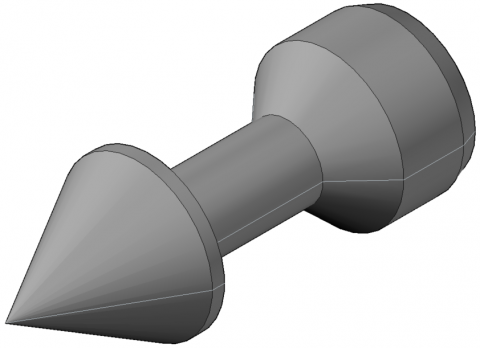(b) Three-dimensional view

## 1c.png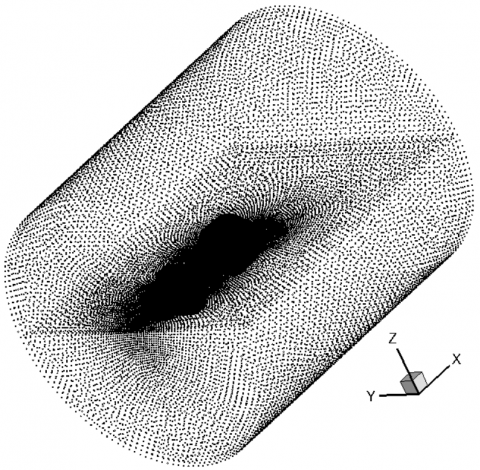(c) The layout of the projectile surrounding points

Figure 1. The structure of the projectile

Figure 2 compares the experimental results with the calculated results. The proposed method in this paper can correctly capture the shock waves on the upper and lower side of the projectile, which are asymmetric under the angle of attack. Figure 3(a) shows the vorticity distribution and partial streamline around the projectile. There was a low-pressure vortex area at the tail of the projectile, with the lowest pressure reaching 10,342Pa. Figure 3(b) shows the temperature and pressure distribution curves of the projectile around the flow field at the z=0 section at the upper and lower walls of the projectile head. It’s found that due to the existence of the angle of attack, the temperature and pressure of the upper wall were higher than those of the lower wall, but in the same change trend. The mixed gas was compressed at the tip of the projectile's head, increasing the temperature and pressure, up to 1,200K. After bypassing the tip of the projectile's head, the temperature and pressure first dropped rapidly within a small distance, the pressure then started to rise and the temperature slowly dropped.

## 2.png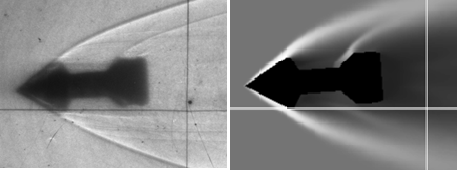Figure 2. Comparison of experimental shadow photos (left) and density cloud images (right)

## 3a.png(a)

## 3b.png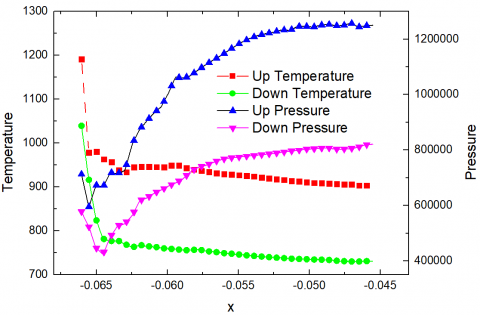(b)

Figure 3. The distribution of vorticity at the x=0 and z=0 sections of the projectile (a), and the temperature and pressure distribution near the upper and lower walls of the head of the projectile at the z=0 section (b)

Figure 4 shows the temperature distribution cloud diagram at z=0 section and y=0. It can be seen that due to the small cone angle d, low flight speed v0, and angle of attack a of the projectile, the shock wave on the head of the projectile was weaker, and the highest gas temperature after the wave was about 1,200K. Located in a small area on the top of the projectile, the chain branching reaction of the chemical reaction cannot be self-accelerated, and thus cannot ignite the methane/air premixed gas to form a stationary oblique detonation.

## 4.pngFigure 4. The temperature distribution cloud diagram at z=0 section (left) and the temperature distribution cloud diagram at y=0 section (right)

4.2 3D muzzle flow field with brake

The brake can reduce the recoil kinetic energy of the gun, and inhibits the muzzle flame to certain degree. In this paper, a meshless method was used to calculate the muzzle flow field of a 12.7mm anti-aircraft machine gun. The muzzle of the machine gun was equipped with an impact brake, ignoring the influence of the moving projectile temporarily in the calculation. The structure of the impact brake mainly includes two orthogonal cylindrical cavities and a baffle, as shown in Figure 5 (a). Considering the symmetry of the flow field, 1/2 flow field was used for calculation. To ensure the accuracy of simulation results, more meshless nodes should be generated around the axis, for a total of 3,285,882 points in the flow field, as shown in Figure 5 (b). The mass fractions of CO, CO2, H2, N2, and H2O in the initial flow field in the muzzle weree 0.4475, 0.26125, 0.0125, 0.12625, and 0.1525, respectively, the pressure was 77.51Mpa, the temperature was 1825.0K, the velocity was 809.5m/s, and the outflow field was static atmosphere.

Figure 6 shows the shadow photo of the muzzle flow field with the brake and the corresponding density cloud map through numerical simulation, which are basically consistent. The flow field structure of the muzzle with a brake was obviously different from that without a brake. The gunpowder gas was discharged through the side hole to form an independent gas jet structure. Its shock wave intersected the jet shock wave of bullet hole to form the muzzle shock wave.

Figures 7 and 8 show the H mass fraction and temperature distribution of the z=0 section at three times t1=185.8μs, t2=380.1μs, and t3=559.9μs, and vorticity distribution diagram of the muzzle flow field at z=0 and x=1.13. From the figures, it can be found that the gunpowder gas has leaked from the bullet holes and side hole; based on the distribution of H mass fraction, a violent chemical reaction occurred first in the brake, forming a secondary combustion in the chamber. At t1, a complete Mach disc, incident shock wave structure and side-hole jet structure were formed. After the oxygen inside the brake was exhausted, the chemical reaction mainly occurred in the jet boundary area in the external flow field, reflecing in the H distribution and temperature distribution. In addition, the shock wave of the bullet hole interacted with that of the side hole, to suppress the bullet hole shock wave, reduce the diameter of the Mach disk, and restrict the middle flame of the muzzle to a certain extent. Over time, a large amount of gunpowder gas mixed and burned with the entrained atmosphere in the side hole jet area. As the gas was evacuated, the size of the bottle-shaped shock structure decreased in the later stage. Figure 9 shows the OH mass fraction distribution and partial streamline of muzzle flow field at x=1.13 and z=0. It can be seen that there was a symmetrical vortex structure in the jet area of the bullet hole and the side hole, forming an entrainment effect. This can promote the progress of chemical reactions and provide more atmosphere. Figure 10 shows the distribution curves of velocity, pressure, temperature, and OH mass fraction on the axis at three times. Due to the existence of the brake, the pressure of the fluid after the bullet hole was reduced, the pressure peaked at the baffle, while the speed dropped to the lowest, and then the speed increased. Then, tthrough the Mach disc, it decreased again, and the Mach disc was shrinking. In addition, the temperature and OH mass fraction distribution curves indicate that the violent chemical reaction area is moving forward, but weakening.

## 5a.png(a)

## 5b.png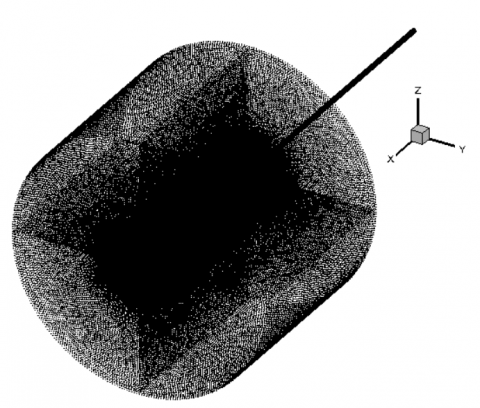(b)

Figure 5. Structure and flow field of the impact brake

## 6.pngFigure 6. Shadow photo of the flow field of the machine gun muzzle and numerically simulated density cloud map

## 7.png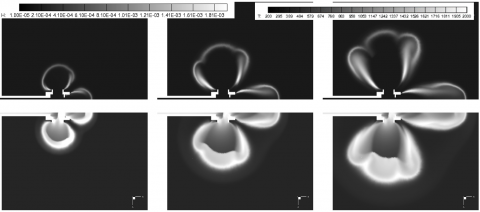Figure 7. The H mass fraction in the muzzle flow field at z=0 (top) and temperature distribution (bottom)

## 8.pngFigure 8. Vorticity distribution diagram of the muzzle flow field at the z=0 and x=1.13 section

## 9a.png(a)

## 9b.png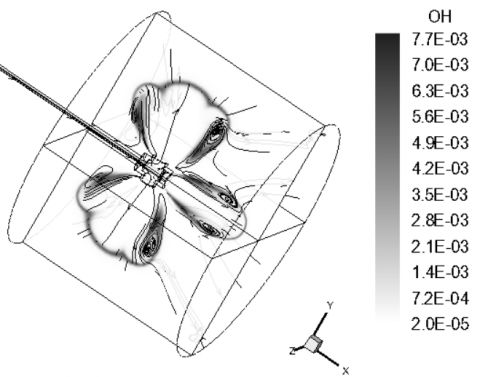(b)

Figure 9. OH mass fraction distribution diagram and partial streamline diagram of the muzzle flow field at x=1.13 and z=0

## 10a.png(a)

## 10b.png(b)

Figure 10. The distribution curve of velocity, pressure, temperature, and OH mass fraction on the axis of the flow field at different times

5. Conclusions

Multi-component high-speed non-equilibrium reaction jets are widely available in practical engineering. This paper proposes the LS meshless method for calculating multi-component high-speed non-equilibrium reaction jets. The multi-component AUFS scheme was used to calculate the convection term, and the finite-rate reaction model updated the flow field parameters under chemical reactions. To solve the rigidity of the chemical reaction in the calculation, the time splitting method was introduced to decouple the items in the governing equation. The 3D flow field was discretized into weighted points with clear meaning and great development potential. In addition, the proposed meshless method was first used to calculated the steady flow around a high-speed flying projectile with an angle of attack. The calculation results were consistent with the experimental results, proving the effectiveness of this algorithm. Then, through a detailed calculation of the 3D muzzle flow field with brakes, it successfully gave the formation process of the complex wave system structure of the muzzle flow field, described the change of the gunpowder gas composites in the time period from the inside of the muzzle to near the muzzle in detail, and explain the influence of the muzzle pressure and the external environment on the formation process of the muzzle flow field and the product composition of the muzzle gas. The research findings provide a new calculation method for the problem of multi-component high-speed non-equilibrium reaction jets.

Acknowledgement

This work is supported by National Natural Science Foundation of China (Grant No.: 51806095, 51906100), Natural Science Foundation of Jiangsu Province (Grant No.: BK2018042206, BK20191015), Research Foundation for the Introduction of Talents in Universities (Grant No.: YKJ201710).

References

 Yee, H.C., Shinn, J.L. (1989). Semi-implicit and fully implicit shock-capturing methods for nonequilibrium flows. AIAA Journal, 27(3): 299-307. https://doi.org/10.2514/3.10112

 Kirk, B., Stogner, R., Oliver, T.A., Bauman, P.T. (2013). Recent advancements in fully implicit numerical methods for hypersonic reacting flows. In 21st AIAA Computational Fluid Dynamics Conference, pp. 2559-2569. https://doi.org/10.2514/6.2013-2559

 Chang, Y.C. (2016). Investigation of skeletal chemical mechanisms for diesel and biodiesel surrogate fuel based on decoupling methodology. Dalian University of Technology.

 Fedkiw, R.P., Merriman, B., Osher, S. (1997). High accuracy numerical methods for thermally perfect gas flows with chemistry. Journal of Computational Physics, 132(2): 175-190.

 da Silva, L.F., Azevedo, J.L., Korzenowski, H. (2000). Unstructured adaptive grid flow simulations of inert and reactive gas mixtures. Journal of Computational Physics, 160(2): 522-540. https://doi.org/10.1006/jcph.2000.6470

 Liu, J., Liu, Y., Zhou, S. (2010). Simulation of shock induced combustion based on a novel uncoupled method. Chinese Journal of Theoretical and Applied Mechanics, 42(3): 572-578.

 Saghafian, A., Terrapon, V.E., Pitsch, H. (2015). An efficient flamelet-based combustion model for compressible flows. Combustion and Flame, 162(3): 652-667. https://doi.org/10.1016/j.combustflame.2014.08.007

 Mei, Z., Shi, C., Fan, X., Wang, X. (2020). Numerical simulation of hypersonic reentry flow Field with gas-phase and surface chemistry models. Materials Today Communications, 22: 100773. https://doi.org/10.1016/j.mtcomm.2019.100773

 Santillana, M., Zhang, L., Yantosca, R. (2016). Estimating numerical errors due to operator splitting in global atmospheric chemistry models: Transport and chemistry. Journal of Computational Physics, 305: 372-386. https://doi.org/10.1016/j.jcp.2015.10.052

 Bussing, T.R., Murman, E.M. (1988). Finite-volume method for the calculation of compressible chemically reacting flows. AIAA Journal, 26(9): 1070-1078. https://doi.org/10.2514/3.10013

 Lee, J.W., Orsini, A., Ruffin, S.M. (2007). Unstructured cartesian-grid methodology for non-equilibrium hypersonic flows. AIAA Paper 2007-548.

 Sussman, M. (1994). A computational study of unsteady shock induced combustion of hydrogen-air mixtures. AIAA Paper 94-3101. https://doi.org/10.2514/6.1994-3101

 Shuen, J.S., Yoon, S. (1989). Numerical study of chemically reacting flows using a lower-upper symmetric successive overrelaxation scheme. AIAA Journal, 27(12): 1752-1760. https://doi.org/10.2514/3.10331

 Sun, W., Gou, X., El-Asrag, H.A., Chen, Z., Ju, Y. (2015). Multi-timescale and correlated dynamic adaptive chemistry modeling of ignition and flame propagation using a real jet fuel surrogate model. Combustion and Flame, 162(4): 1530-1539. https://doi.org/10.1016/j.combustflame.2014.11.017

 Gou, X., Sun, W., Chen, Z., Ju, Y. (2010). A dynamic multi-timescale method for combustion modeling with detailed and reduced chemical kinetic mechanisms. Combustion and Flame, 157(6): 1111-1121. https://doi.org/10.1016/j.combustflame.2010.02.020

 Liu, G.R. (2009). Meshfree methods: moving beyond the finite element method. Taylor & Francis.

 Jiang, T., Chen, Z.C., Lu, W.G., Yuan, J.Y., Wang, D.S. (2018). An efficient split-step and implicit pure mesh-free method for the 2D/3D nonlinear Gross–Pitaevskii equations. Computer Physics Communications, 231: 19-30. https://doi.org/10.1016/j.cpc.2018.05.007

 Idelsohn, S.R., Onate, E., Calvo, N., Del Pin, F. (2003). The meshless finite element method. International Journal for Numerical Methods in Engineering, 58(6): 893-912. https://doi.org/10.1002/nme.798

 Perrone, N., Kao, R. (1975). A general finite difference method for arbitrary meshes. Computers & Structures, 5(1): 45-57. https://doi.org/10.1016/0045-7949(75)90018-8

 Liszka, T., Orkisz, J. (1980). The finite difference method at arbitrary irregular grids and its application in applied mechanics. Computers & Structures, 11(1-2): 83-95. https://doi.org/10.1016/0045-7949(80)90149-2

 Ku, C.Y., Liu, C.Y., Yeih, W., Liu, C.S., Fan, C.M. (2019). A novel space–time meshless method for solving the backward heat conduction problem. International Journal of Heat and Mass Transfer, 130: 109-122. https://doi.org/10.1016/j.ijheatmasstransfer.2018.10.083

 Ahmad, I., Riaz, M., Ayaz, M., Arif, M., Islam, S., Kumam, P. (2019). Numerical simulation of partial differential equations via local meshless method. Symmetry, 11(2): 257. https://doi.org/10.3390/sym11020257

 Depolli, M., Kosec, G. (2017). Assessment of differential evolution for multi-objective optimization in a natural convection problem solved by a local meshless method. Engineering Optimization, 49(4): 675-692. https://doi.org/10.1080/0305215X.2016.1197686

 Löhner, R., Sacco, C., Onate, E., Idelsohn, S. (2002). A finite point method for compressible flow. International Journal for Numerical Methods in Engineering, 53(8): 1765-1779. https://doi.org/10.1002/nme.334

 Ortega, E., Oñate, E., Idelsohn, S. (2009). A finite point method for adaptive three‐dimensional compressible flow calculations. International Journal for Numerical Methods in Fluids, 60(9): 937-971. https://doi.org/10.1002/fld.1892

 Erhart, K.J., Gerace, S.A., Divo, E.A., Kassab, A.J. (2009). An RBF interpolated generalized finite difference meshless method for compressible turbulent flows. In ASME International Mechanical Engineering Congress and Exposition, 43826: 571-581. https://doi.org/10.1115/IMECE2009-11452

 Munikrishna, N., Balakrishnan, N. (2011). Turbulent flow computations on a hybrid cartesian point distribution using meshless solver LSFD-U. Computers & Fluids, 40(1): 118-138. https://doi.org/10.1016/j.compfluid.2010.08.017

 Gargari, S.F., Kolahdoozan, M., Afshar, M.H. (2018). Mixed Discrete Least Squares Meshfree method for solving the incompressible Navier–Stokes equations. Engineering Analysis with Boundary Elements, 88: 64-79. https://doi.org/10.1016/j.enganabound.2017.12.018

 Gargari, S.F., Kolahdoozan, M., Afshar, M.H., Dabiri, S. (2019). An Eulerian–Lagrangian mixed discrete least squares meshfree method for incompressible multiphase flow problems. Applied Mathematical Modelling, 76: 193-224. https://doi.org/10.1016/j.apm.2019.06.002

 Li, Z., Ferrarotti, M., Cuoci, A., Parente, A. (2018). Finite-rate chemistry modelling of non-conventional combustion regimes using a Partially-Stirred Reactor closure: Combustion model formulation and implementation details. Applied Energy, 225: 637-655. https://doi.org/10.1016/j.apenergy.2018.04.085

 Sun, M., Takayama, K. (2003). An artificially upstream flux vector splitting scheme for the Euler equations. Journal of Computational Physics, 189(1): 305-329. https://doi.org/10.1016/S0021-9991(03)00212-2

 Wang, L., Xu, H.Q., Wu, W., Xue, R. (2016). A novel gridless adaptive method for simulating unsteady flows. Journal of Aerodynamics, (4): 497-502.

 Dai, S.L., Xu, H.Q., Wang, B. (2009). Numerical simulation of secondary muzzle flash including high-speed projectile using parallel computation method. Journal of Ballistics, (1): 83-86.